# MBQN vignette

This package contains a modified quantile normalization (QN) for preprocessing and analysis of omics or other matrix-like organized data with intensity values biased by global, columnwise distortions of intensity mean and scale. The modification balances the mean intensity of features (rows) which are rank invariant (RI) or nearly rank invariant (NRI) across samples (columns) before quantile normalization . This helps to prevent an over-correction of the intensity profiles of RI and NRI features by classical QN and therefore supports the reduction of systematics in downstream analyses. Additional package functions help to detect, identify, and visualize potential RI or NRI features in the data and demonstrate the use of the modification.

## Installation

To install this package, you need R version >= 3.6.

For installation from Bioconductor run in R:

# if (!requireNamespace("BiocManager", quietly = TRUE))
#    install.packages("BiocManager")
BiocManager::install("MBQN")

## Dependencies

The core of the MBQN package uses normalizeQuantiles() from the package limma , available at https://bioconductor.org/packages/release/bioc/html/limma.html, for computation of the quantile normalization. Optionally, normalize.quantiles() from the package preprocessCore , available at https://bioconductor.org/packages/release/bioc/html/preprocessCore.html, can be used.

The function getPXDfile() in MBQN uses data from the PRIDE repository. To run this function one needs the R package rpx  to download the data.

To install these packages in R run:

# if (!requireNamespace("BiocManager", quietly = TRUE))
#    install.packages("BiocManager")
# BiocManager::install(pkgs = c("preprocessCore","limma","rpx","SummarizedExperiment"))

## Usage

The package provides two basic functions: mbqn() applies QN or mean/median-balanced quantile normalization (MBQN) to a matrix. mbqnNRI() applies quantile normalization and mean/median-balanced quantile normalization only to selected NRI and RI features, specified by a threshold or manually. The input matrix may contain NAs. To run one of these functions you will need to provide an input matrix (see Examples). The argument FUN is used to select between classical quantile normalization (default), and mean or median balanced quantile normalization. The function mbqnGetNRIfeatures() and mbqnPlotRI() can be used to check a data matrix for RI or NRI features. They provide a list of potential RI/NRI features together with their rank invariance frequency and a graphical output.

## Examples

Example 1: Generate a distorted omics-like matrix of log2-transformed intensities with missing values and a single rank invariant feature:

## basic example
library("MBQN")
set.seed(1234)
# data generation
mtx <- mbqnSimuData("omics.dep")
# data distortion
mtx <- mbqnSimuDistortion(mtx)$x.mod plot.new() mbqnBoxplot(mtx, irow = 1, main = "Unnormalized") ## Warning in (function (z, notch = FALSE, width = NULL, varwidth = FALSE, : ## Duplicated argument main = "Unnormalized" is disregarded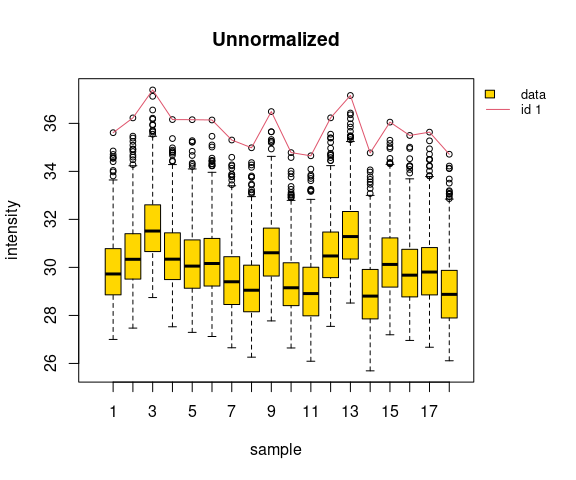Fig. 1 Boxplot of the unnormalized, distorted intensity data matrix. The first feature is an RI feature (red line). It has maximum intensity for each sample! Apply check for rank invariant (RI) or nearly rank invariant (NRI) features to the data matrix and visualize result: res <- mbqnGetNRIfeatures(mtx, low_thr = 0.5) ## Caution: There might be multiple RI/NRI features! ## Maximum frequency of RI/NRI feature(s): 100 % Apply quantile normalization with and without balancing the RI feature and compare the intensity features: plot.new() mbqn.mtx <- mbqnNRI(x = mtx, FUN = median, verbose = FALSE) # MBQN qn.mtx <- mbqnNRI(x = mtx, FUN = NULL, verbose = FALSE) # QN mbqnBoxplot(mbqn.mtx, irow = res$ip, vals = data.frame(QN = qn.mtx[res$ip,]), main = "Normalized") ## Warning in (function (z, notch = FALSE, width = NULL, varwidth = FALSE, : ## Duplicated argument main = "Normalized" is disregarded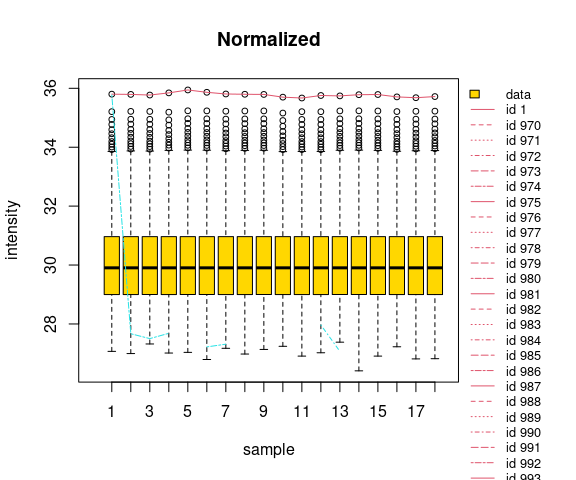Fig. 2 Quantile normalized intensities with balanced and unbalanced normalized RI feature. Classical quantile normalization suppresses any intensity variation of the RI feature, while the MBQN preserves its variation while reducing systematic batch effects! Example 2: Visualize the effect of normalization on rank mixing and rank invariant intensity features by comparing the intensity distribution of unnormalized, quantile and mean/median-balanced quantile normalized data on a matrix where rows represent features, e.g. of protein abundances/intensities, and columns represent samples. ## basic example library("MBQN") set.seed(1234) mtx <- mbqnSimuData("omics.dep") # Alternatively: mtx <- matrix( # c(5,2,3,NA,2,4,1,4,2,3,1,4,6,NA,1,3,NA,1,4,3,NA,1,2,3),ncol=4) Perform QN, median balanced QN, and QN with median balanced NRI feature. qn.mtx <- mbqn(mtx,FUN=NULL, verbose = FALSE) mbqn.mtx <- mbqn(mtx,FUN = "median", verbose = FALSE) qn.nri.mtx <- mbqnNRI(mtx,FUN = "median", low_thr = 0.5, verbose = FALSE) Check saturation i.e. for rank invariance. res <- mbqnGetNRIfeatures(mtx, low_thr = 0.5) ## Caution: There might be multiple RI/NRI features! ## Maximum frequency of RI/NRI feature(s): 100 % # Maximum frequency of RI/NRI feature(s): 100 % Example 3: Apply a two-sided t-test before and after application of different normalizations to a simulated, differentially expressed and distorted RI feature. The feature is obtained from a simulated dataset where each sample is distorted in mean and scale. #plot.new() mtx <- mbqnSimuData("omics.dep", show.fig = FALSE) mod.mtx <- mbqnSimuDistortion(mtx, s.mean = 0.05, s.scale = 0.01) mtx2 <- mod.mtx mod.mtx <- mod.mtx$x.mod

res <- mbqnGetNRIfeatures(mod.mtx, low_thr = 0.5)
## Caution: There might be multiple RI/NRI features!
## Maximum frequency of RI/NRI feature(s):  100 %
# undistorted feature
feature1 <- mtx[1,]
# distorted feature
feature1mod = mod.mtx[1,]
# feature after normalization
qn.feature1 = mbqn(mod.mtx, verbose = FALSE)[1,]
qn.mtx = mbqn(mod.mtx,verbose = FALSE)

mbqn.mtx = mbqn(mod.mtx, FUN = "mean",verbose = FALSE)
mbqn.feature1 = mbqn(mod.mtx, FUN = "mean",verbose = FALSE)[1,]

Apply t-test:

# undistorted feature
ttest.res0 <- t.test(feature1[seq_len(9)], feature1[c(10:18)],
var.equal =TRUE)
# distorted feature
ttest.res1 <- t.test(feature1mod[seq_len(9)], feature1mod[c(10:18)],
var.equal =TRUE)
# mbqn normalized distorted feature
ttest.res <- t.test(mbqn.feature1[seq_len(9)], mbqn.feature1[c(10:18)],
var.equal =TRUE)

Compare QN, MBQN and original feature.

plot.new()
matplot(t(rbind(feature1 = feature1,
mod.feature1 = (feature1mod-mean(feature1mod))/25+mean(feature1),
qn.feature1 = (qn.feature1-mean(qn.feature1))+mean(feature1),
mbqn.feature1 = (
mbqn.feature1-mean(mbqn.feature1))+mean(feature1))),
type = "b", lty = c(1,1,1), pch = "o",
ylab = "intensity",
xlab = "sample",
main = "Differentially expressed RI feature",
ylim = c(34.48,34.85))
legend(x=11,y= 34.86, legend = c("feature","distorted feature/25" ,
"QN feature", " MBQN feature"),pch = 1,
col = c(1,2,3,4), lty= c(1,1,1,1), bty = "n", y.intersp = 1.5,
x.intersp = 0.2)
legend(x = .1, y = 34.6,
legend = paste("p-value (t-test) =",round(ttest.res1$p.value,2), "\np-value (t-test, mbqn) =", round(ttest.res$p.value,4)),
bty = "n", x.intersp = 0)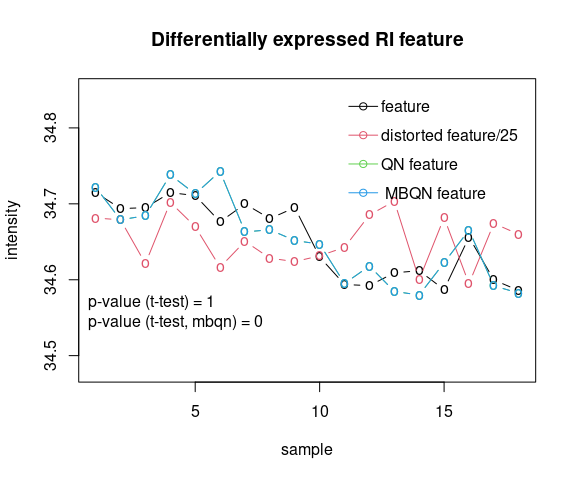Fig. 3

if (ttest.res$p.value<0.05) message("H0 (=equal mean) is rejected!") ## H0 (=equal mean) is rejected! # print(mtx2$x.mod)
# print(mtx2$mx.offset) # print(mtx2$mx.scale)
print(paste("ttest.undistorted =",ttest.res0)) 
##   "ttest.undistorted = c(t = 10.0729609396921)"
##   "ttest.undistorted = c(df = 16)"
##   "ttest.undistorted = 2.48237068197741e-08"
##   "ttest.undistorted = c(0.0715256392245863, 0.109656286984875)"
##   "ttest.undistorted = c(mean of x = 34.6978790054118, mean of y = 34.607288042307)"
##   "ttest.undistorted = c(difference in means = 0)"
##   "ttest.undistorted = 0.00899347904227051"
##   "ttest.undistorted = two.sided"
##   "ttest.undistorted =  Two Sample t-test"
##  "ttest.undistorted = feature1[seq_len(9)] and feature1[c(10:18)]"
print(paste("ttest.distorted =", ttest.res1))
##   "ttest.distorted = c(t = -0.00510882259762921)"
##   "ttest.distorted = c(df = 16)"
##   "ttest.distorted = 0.995986923648603"
##   "ttest.distorted = c(-0.877522816321726, 0.873303448226306)"
##   "ttest.distorted = c(mean of x = 36.1994057861729, mean of y = 36.2015154702206)"
##   "ttest.distorted = c(difference in means = 0)"
##   "ttest.distorted = 0.412949169283929"
##   "ttest.distorted = two.sided"
##   "ttest.distorted =  Two Sample t-test"
##  "ttest.distorted = feature1mod[seq_len(9)] and feature1mod[c(10:18)]"
print(paste("ttest.mbqndistorted =", ttest.res))
##   "ttest.mbqndistorted = c(t = 5.61617252905213)"
##   "ttest.mbqndistorted = c(df = 16)"
##   "ttest.mbqndistorted = 3.86388948816137e-05"
##   "ttest.mbqndistorted = c(0.0534488302470274, 0.118264504238475)"
##   "ttest.mbqndistorted = c(mean of x = 36.2430019696062, mean of y = 36.1571453023635)"
##   "ttest.mbqndistorted = c(difference in means = 0)"
##   "ttest.mbqndistorted = 0.0152873984548409"
##   "ttest.mbqndistorted = two.sided"
##   "ttest.mbqndistorted =  Two Sample t-test"
##  "ttest.mbqndistorted = mbqn.feature1[seq_len(9)] and mbqn.feature1[c(10:18)]"

Example 4: This example downloads an LFQ intensity dataset from the PRIDE repository, normalizes the data, identifies RI/NRI features, and give graphical output. One can choose between four data sets. By default data files are stored in the current working directory currentdir/PXDxxx.

The following example illustrates normalization of LFQ intensity data from the PRIDE repository. For illustration purpose, we use the data set “PXD001584” (file size 10.8 MB)

Other data examples are
* “PXD005138” - contains one RI feature (file size 7440.6 MB)
* “PXD005861” - contains one RI feature (file size 334.7 MB)
* “PXD006617” - contains one RI feature (file size 290.8 MB)

library("SummarizedExperiment")
## Loading required package: MatrixGenerics
## Loading required package: matrixStats
##
## Attaching package: 'MatrixGenerics'
## The following objects are masked from 'package:matrixStats':
##
##     colAlls, colAnyNAs, colAnys, colAvgsPerRowSet, colCollapse,
##     colCounts, colCummaxs, colCummins, colCumprods, colCumsums,
##     colDiffs, colIQRDiffs, colIQRs, colLogSumExps, colMadDiffs,
##     colMads, colMaxs, colMeans2, colMedians, colMins, colOrderStats,
##     colProds, colQuantiles, colRanges, colRanks, colSdDiffs, colSds,
##     colSums2, colTabulates, colVarDiffs, colVars, colWeightedMads,
##     colWeightedMeans, colWeightedMedians, colWeightedSds,
##     colWeightedVars, rowAlls, rowAnyNAs, rowAnys, rowAvgsPerColSet,
##     rowCollapse, rowCounts, rowCummaxs, rowCummins, rowCumprods,
##     rowCumsums, rowDiffs, rowIQRDiffs, rowIQRs, rowLogSumExps,
##     rowOrderStats, rowProds, rowQuantiles, rowRanges, rowRanks,
##     rowSdDiffs, rowSds, rowSums2, rowTabulates, rowVarDiffs, rowVars,
##     rowWeightedSds, rowWeightedVars
## Loading required package: GenomicRanges
## Loading required package: stats4
## Loading required package: BiocGenerics
## Loading required package: parallel
##
## Attaching package: 'BiocGenerics'
## The following objects are masked from 'package:parallel':
##
##     clusterApply, clusterApplyLB, clusterCall, clusterEvalQ,
##     clusterExport, clusterMap, parApply, parCapply, parLapply,
##     parLapplyLB, parRapply, parSapply, parSapplyLB
## The following objects are masked from 'package:stats':
##
##     IQR, mad, sd, var, xtabs
## The following objects are masked from 'package:base':
##
##     Filter, Find, Map, Position, Reduce, anyDuplicated, append,
##     as.data.frame, basename, cbind, colnames, dirname, do.call,
##     duplicated, eval, evalq, get, grep, grepl, intersect, is.unsorted,
##     lapply, mapply, match, mget, order, paste, pmax, pmax.int, pmin,
##     pmin.int, rank, rbind, rownames, sapply, setdiff, sort, table,
##     tapply, union, unique, unsplit, which.max, which.min
## Loading required package: S4Vectors
##
## Attaching package: 'S4Vectors'
## The following objects are masked from 'package:base':
##
##     I, expand.grid, unname
## Loading required package: IRanges
## Loading required package: GenomeInfoDb
## Loading required package: Biobase
## Welcome to Bioconductor
##
##     Vignettes contain introductory material; view with
##     'browseVignettes()'. To cite Bioconductor, see
##     'citation("Biobase")', and for packages 'citation("pkgname")'.
##
## Attaching package: 'Biobase'
## The following object is masked from 'package:MatrixGenerics':
##
##     rowMedians
## The following objects are masked from 'package:matrixStats':
##
##     anyMissing, rowMedians
pxd_id <- "PXD001584"

#getPXDfile(pxd_id)

out <- mbqnLoadFile(pxd_id, file.pattern = "proteinGroups.txt")
## File does not exist - proceed with download...
##  "ftp://ftp.pride.ebi.ac.uk/pride/data/archive/2015/01/PXD001584"
## using temporary cache /tmp/Rtmpwy7HOG/BiocFileCache
##  "ftp://ftp.pride.ebi.ac.uk/pride/data/archive/2015/01/PXD001584"
## Number of proteins with empty entries: 0
# filter for potential contaminants and identified only by site features
out <- out[!rowData(out)[["ixs"]],]

# extract data and feature annotation
mtx <- assays(out)[["data"]]
featureAnnotations <- rowData(out)

low_thr <- 0.5
ylim <- NULL
ix <- seq_len(ncol(mtx))

ix <- c(1:9,19:27)
mtx <- mtx[,ix]
ylim.qn <- ylim <- c(22.5,36)

####################################################################################
plot.new()
res <- mbqnPlotAll(mtx,
FUN = median,
low_thr = low_thr,
las = 2,
type = "l",
feature_index = NULL,
show_nri_only = TRUE,
axis.cex = 0.5,
y.intersp= 0.5)
## Caution: There might be multiple RI/NRI features!
## Maximum frequency of RI/NRI feature(s):  100 %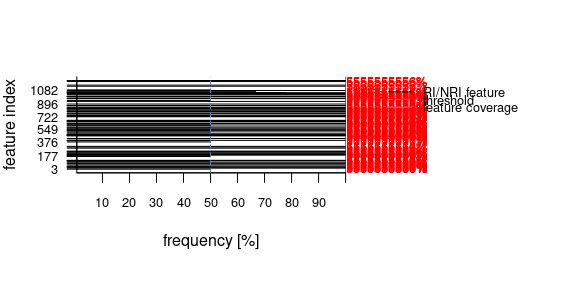Fig. 4 Correctly detected and identified RI (100 %RI) and a NRI feature (75 %RI) each with a data coverage of 100% across samples.

#dev.off()

# get protein name of strongest nri/ri feature
nri_max <- as.numeric(names(which.max(res$nri))) #featureAnnotations$proteinDescription[nri_max]
#featureAnnotations$proteinName[nri_max] #featureAnnotations$nbPeptides[nri_max]

colnames(mtx) <- gsub("LFQ intensity","",colnames(mtx))
mbqn.mtx <- mbqn(mtx,FUN = median)
qn.mtx <- mbqn(mtx,FUN = NULL)

# Boxplot of QN intensity features, highlight RI/NRI Features
if(length(ylim)==0) {
ylim <- c(floor(min(range(mbqn.mtx, na.rm = TRUE))),ceiling(max(range(mbqn.mtx, na.rm = TRUE))))
ylim.qn <- c(floor(min(range(qn.mtx, na.rm = TRUE))),ceiling(max(range(mbqn.mtx, na.rm = TRUE))))
}

df <- data.frame(qn.mtx[as.numeric(names(res$nri)),]) if(ncol(df)==1) df <- t(df) rownames(df) <- paste("QN feature",names(res$nri))
df2 <- data.frame(mtx[as.numeric(names(res$nri)),]) if(ncol(df2)==1) df2 <- t(df2) rownames(df2) <- paste("unnormal. feature",names(res$nri))
colnames(df) <- colnames(df2)
df <- rbind(df,df2)
df <- as.data.frame(t(df))
  mtx.nri <- mbqnNRI(mtx,FUN = median,low_thr = 0.5, verbose = FALSE)

plot.new()
mbqnBoxplot(mtx = mtx.nri,
irow = as.numeric(names(res\$nri)),
ylim = ylim.qn, xlab= "", las=2,
ylab = "LFQ intensity",
vals = df,lwd = 1.,
main = "QN with RI/NRI balanced",
cex.axis = 1, cex.lab = .9, cex = .9, y.intersp = 0.5)
## Warning in (function (z, notch = FALSE, width = NULL, varwidth = FALSE, :
## c("Duplicated arguments list(xlab = \"\", las = 2, ylab = \"LFQ intensity\",
## main = \"QN with RI/NRI balanced\", are disregarded", "Duplicated arguments cex
## = 0.9) are disregarded")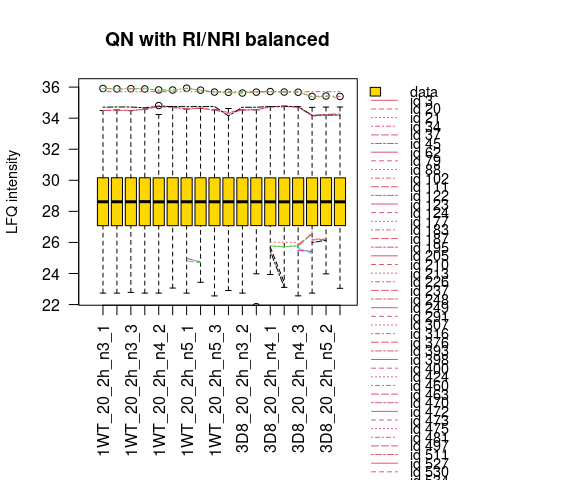Fig. 5

## Documentation

To view the documentation for MBQN, type in R

??MBQN